# Chemical Equilibrium Formula

Chemical Equilibrium Formula

During the initial moments of a chemical reaction, the reactants collide and form products. As the reaction continues the amount of product increases and in certain situations, can turn back into reactants. When the rate of the forward reaction is equal to the rate of the reverse reaction, chemical equilibrium is established.

In a reaction that has reached equilibrium, a constant can be calculated using the law of mass action. The law of mass action uses a ratio of products over reactants with the following setup.

In the reaction: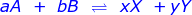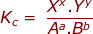where:

Kc = the equilibrium constant using the molar concentrations of reactants and products

Xx and Yy are the product concentrations raised to the power of their coefficients

Aa and Bb are the reactant concentrations raised to the power of their coefficients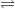signifies the reaction is at equilibrium

Chemical Equilibrium Formula Questions:

1. Calculate the equilibrium constant, Kc, for the following reaction where [N2] = 1.5 M, [H2] = 2.4 M and [NH3] = 3.8 M.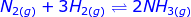The brackets surrounding a reactant or product (for example:[N2]) signifies a molar concentration.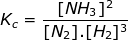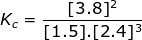Kc = 0.70

2. Calculate the equilibrium constant, Kc, for the following reaction where [O2] = 2.0 M and [O3] = 1.7 M.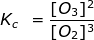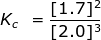Kc = 0.36

 Related Links: Chemical Equilibrium Quiz Chemical Equilibrium Quiz Solubility and Acid-Base Equilibrium Quiz Chemical Reaction Formula Chemical Formula